## Eugenie Hunsicker, Hengguang Li, Victor Nistor and Ville Uski: Analysis of Schrödinger operators with inverse square potentials I: regularity results in 3D, p.157-178

### Abstract:

Let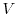be a potential on ℜ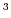that is smooth everywhere except at a discrete set S of points, where it has singularities of the form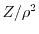, with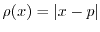for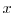close to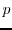and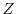continuous on ℜwith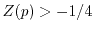for p ∈ S. Also assume that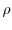andare smooth outside S andis smooth in polar coordinates around each singular point. We either assume thatis periodic or that the set S is finite andextends to a smooth function on the radial compactification of ℜthat is bounded outside a compact set containing S. In the periodic case, we let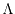be the periodicity lattice and define T: = ℜ3/Λ. We obtain regularity results in weighted Sobolev space for the eigenfunctions of the Schrödinger-type operator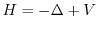acting on L2(T), as well as for the induced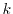-Hamiltonians Hk obtained by restricting the action of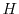to Bloch waves. Under some additional assumptions, we extend these regularity and solvability results to the non-periodic case. We sketch some applications to approximation of eigenfunctions and eigenvalues that will be studied in more detail in a second paper.

Key Words: Regularity of eigenfunctions, Schrodinger operator, eigenvalue approximations, inverse square potential, regularity, weighted Sobolev spaces, rate of convergence of numerical methods, solid state physics.

2000 Mathematics Subject Classification: Primary: 35B65;
Secondary: 35J10, 65N12, 81Q05, 35B10, 35P10.